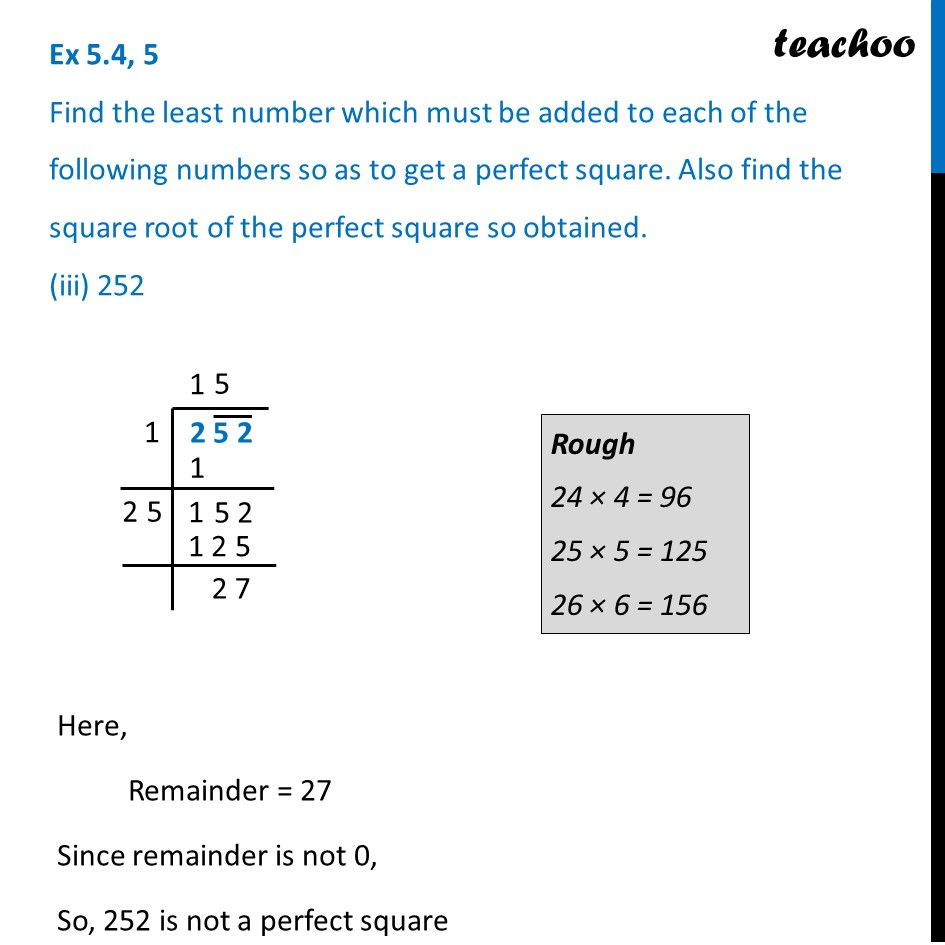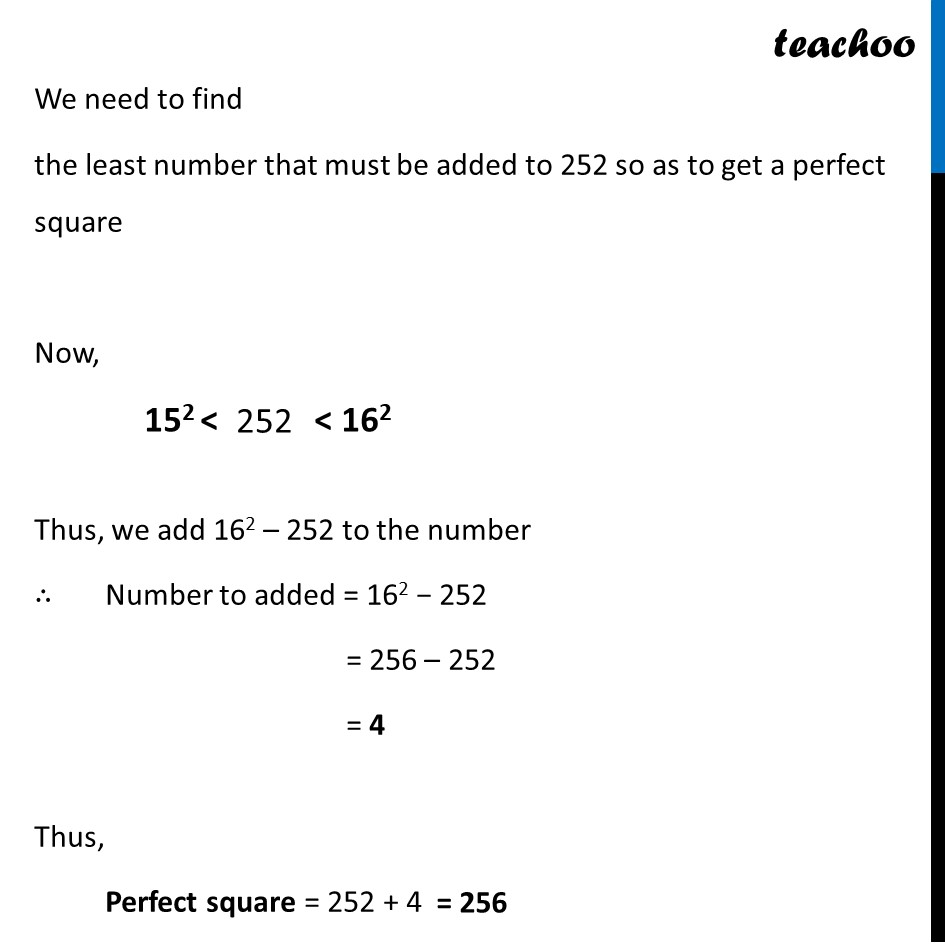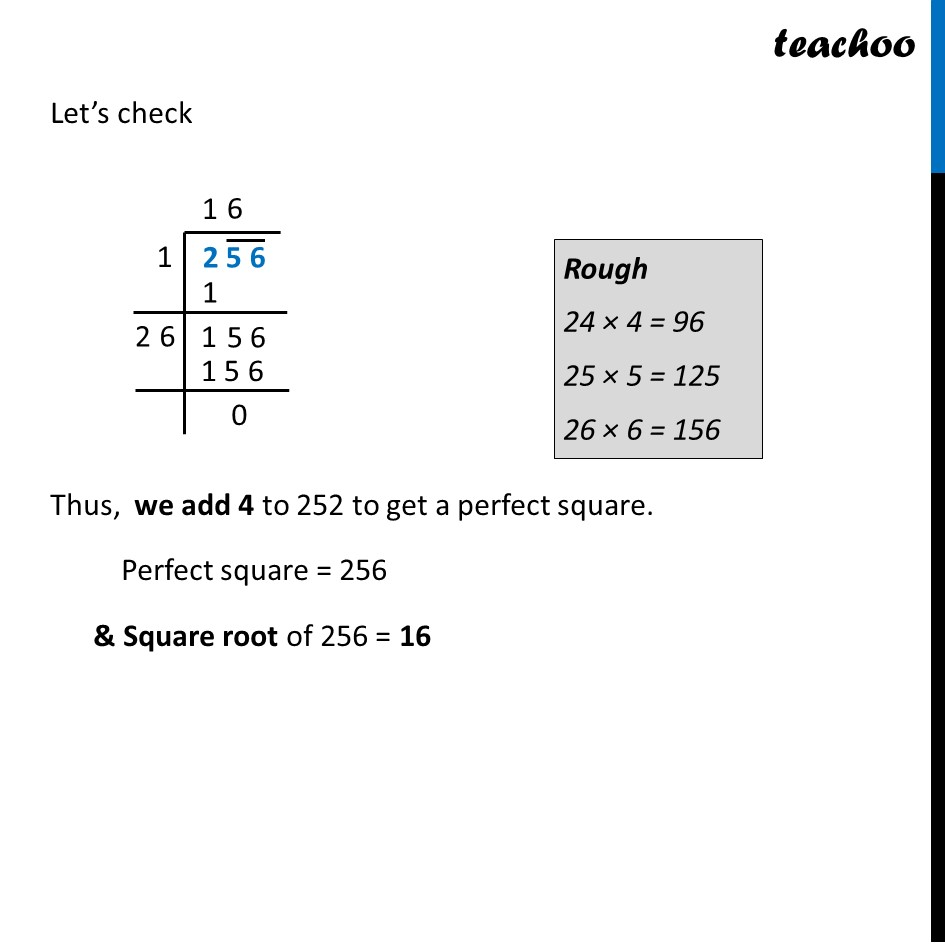Ex 5.4

Chapter 5 Class 8 Squares and Square Roots
Serial order wiseLearn in your speed, with individual attention - Teachoo Maths 1-on-1 Class

### Transcript

Ex 5.4, 5 Find the least number which must be added to each of the following numbers so as to get a perfect square. Also find the square root of the perfect square so obtained. (iii) 252 Here, Remainder = 27 Since remainder is not 0, So, 252 is not a perfect square We need to find the least number that must be added to 252 so as to get a perfect square Now, Thus, we add 162 – 252 to the number ∴ Number to added = 162 − 252 = 256 – 252 = 4 Thus, Perfect square = 252 + 4 Let’s check Thus, we add 4 to 252 to get a perfect square. Perfect square = 256 & Square root of 256 = 16